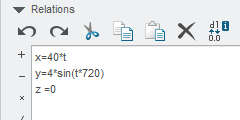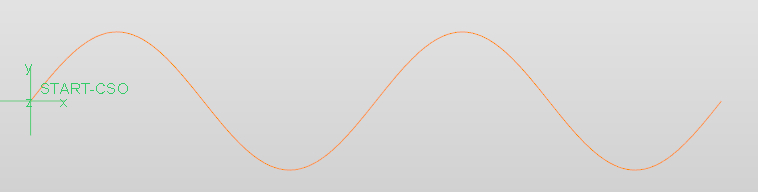cancel
Showing results for
Did you mean:
cancel
Showing results for
Did you mean:

SOLVED

## Need to build repetitive curve in a sketch

I am needing to build a sketch that the curve needs to follow an exact pattern.  I have attached a sketch that shows the basic curvature. (As it is freehand the shape is not exact however)The pattern of curvature needs to follow a point placement like this.  The initial point is at X=0 and Y=+.06.  The second point on the curve would be X=+.5 and Y=-.06.  The 3rd point on the curve would be X=1 and Y=.06.  Etc...

I want to build a curve where I don't have to manually enter the dimensions as the size will extend for close to 50 inches.

My initial thought would be that Relations might be the way to go, but I have no experience with this and don't know if it would be capable of what I am looking to do.

Tags (1)
1 ACCEPTED SOLUTION

Accepted Solutions

## Re: Need to build repetitive curve in a sketch

I'll try again tomorrow. Trying to remember the formula just now.

X= 40*(t*720)
Y= 4*sin*(t*360)
Z=0

In this case 40 is the wavelength.
720 is 2 cycles
I think 4 is amplitude for y
Z is zero because it's in a single plane.

Difficult when not in front of a car screen to check.
10 REPLIES 10

## Re: Need to build repetitive curve in a sketch

Still looking this over online.  I haven't pieced this together but I am now wondering whether this can be done as a relation at all within a sketch.

I am seeing examples how using a variable section sweep can influence different shapes but haven't seen a simple straight line oscillating curve that would result in the basic sweep shape of the curve on my initial post.

## Re: Need to build repetitive curve in a sketch

HI, Use curve from equation.

Datum\Curve\curve from equation. Example can be tailored from below.## Re: Need to build repetitive curve in a sketch

Rcrerar-2:  The images you attached aren't showing.  I will see if I can find the location you are suggesting but if there is an easy way to see these images it would be great.

## Re: Need to build repetitive curve in a sketch

I'll try again tomorrow. Trying to remember the formula just now.

X= 40*(t*720)
Y= 4*sin*(t*360)
Z=0

In this case 40 is the wavelength.
720 is 2 cycles
I think 4 is amplitude for y
Z is zero because it's in a single plane.

Difficult when not in front of a car screen to check.

## Re: Need to build repetitive curve in a sketch

This is excellent!

I just did find a video that showed roughly what I needed and proved this out.

I will check to see how your numbers differentiate.

This is a little fudgy so it may be that your numbers are closer in line than the formula I was using.

## Re: Need to build repetitive curve in a sketch

Just Checked the formula I used.

x=50*t

y= 4*sin(t*720)

z=0

curve would be 50 units long and total height is 8 units crest to crest. This produces 2 cycles if you want more then each cycle is multiple of 360.

If it is not a sin curve then the maths needs working out.

Have fun.

## Re: Need to build repetitive curve in a sketch

Subsitute 'sin' for 'cos' in the formula & you get another variation of the curve.

Worth a mention.

## Re: Need to build repetitive curve in a sketch

rcrerar:  Here is the formula that I went with.  This worked great.I will be able to use this pattern in the straight stretches, but unfortunately I can not use this for twisting around a large radii in our die.  With that I will be developing a different technique involving a pattern and some fillets.

When you extrude this feature it looks a lot like a sun chip.  Unfortunately I can't bend this feature at a specific large radii while maintaining the tilt I will need.

John:  I did for a while consider the cosine function for doing a bend, but in the end I knew it would be a bear to maintain a specific bend radii as well being unable to carry through the proper inclination.

In the end I know that I can still use this for the straight stretches in my die.  It gives a beautiful result.

Highlighted

## Re: Need to build repetitive curve in a sketch

"I will be able to use this pattern in the straight stretches, but unfortunately I can not use this for twisting around a large radii in our die.  With that I will be developing a different technique involving a pattern and some fillets."

I am not sure what you mean exactly by your statement but would the attached image help?